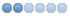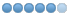# 7i96 configuration Tool and Gantry XYZ Machines

• SSIRon
• Offline
• Junior Member
•02 Jan 2022 23:45 #230593 by SSIRon
i have used the 7i96 Config Tool and did get a machine running while connect to the board but not to stepper just yet. also i can movement on xy and z but not the forth axis.  I have a gantry machine XXYZ and i have searched high and low for examples of this configuration but nothing comes close to the ini or hal files that are generated by the tool.  I see in the example that the gantry is yy or xx but not xu or yu as i would expect.  Yet if i try to remove a reference to the u axis linuxcnc fails.  Attached are my ini and hal files.

#### File Attachment:

File Name: mesarouter.hal
File Size:7 KB

#### File Attachment:

File Name: mesarouter.ini
File Size:4 KB
##### Attachments:

• RNJFAB
• Offline
• Elite Member
•03 Jan 2022 02:11 - 03 Jan 2022 02:17 #230605 by RNJFAB
Hey SSIRon,

I'm no expert, they will be around shortly.

I found when I set up my machine it created a y & y1 axis for the gantry. these were then linked in the ini file.

I have attached a example of it below. This was all created when setting up the machine in the config. I made sure I put a sticker on each axis motor so I knew when adjusting limits which motor I was adjusting. Its amazing how quickly you can get lost in the CNC maze.

I would re-do the config and set up your machine with a y & y1 or a x & x1.

********************************************
[AXIS_Y]
[JOINT_1]
TYPE = LINEAR
HOME = 0.0
FERROR = 10.0
MIN_FERROR = 1.0
MAX_VELOCITY = 60.0
MAX_ACCELERATION = 550.0
MAX_ACCELERATION
STEPGEN_MAXVEL = 70
STEPGEN_MAXACCEL = 940
P = 1000.0
I = 0.0
D = 0.0
FF0 = 0.0
FF1 = 1.0
FF2 = 0.0
BIAS = 0.0
MAX_OUTPUT = 0.0
DIRSETUP = 10000
DIRHOLD = 10000
STEPLEN = 10000
STEPSPACE = 10000
STEP_SCALE = -160
MIN_LIMIT = -10
MAX_LIMIT = 1080.01
HOME_OFFSET = -1
HOME_SEARCH_VEL = -25
HOME_LATCH_VEL = -5
HOME_FINAL_VEL = 15
HOME_IGNOR_LIMITS = YES
HOME_SEQUENCE = -1

[JOINT_2]
TYPE = LINEAR
HOME = 0.0
FERROR = 10.0
MIN_FERROR = 1.0
MAX_VELOCITY = 60.0
MAX_ACCELERATION = 550.0
MAX_ACCELERATION
STEPGEN_MAXVEL = 70
STEPGEN_MAXACCEL = 940
P = 1000.0
I = 0.0
D = 0.0
FF0 = 0.0
FF1 = 1.0
FF2 = 0.0
BIAS = 0.0
MAX_OUTPUT = 0.0
DIRSETUP = 10000
DIRHOLD = 10000
STEPLEN = 10000
STEPSPACE = 10000
STEP_SCALE = -160
MIN_LIMIT = -10
MAX_LIMIT = 1080.01
HOME_OFFSET = -1
HOME_SEARCH_VEL = -25
HOME_LATCH_VEL = -5
HOME_FINAL_VEL = 15
HOME_IGNOR_LIMITS = YES
HOME_SEQUENCE = -1
******************************************
Last edit: 03 Jan 2022 02:17 by RNJFAB.

• SSIRon
• Offline
• Junior Member
•03 Jan 2022 02:23 #230607 by SSIRon
thank you - this is a odd as this is not what i am getting but i am going to try it again and see what i get. back shortly

• SSIRon
• Offline
• Junior Member
•03 Jan 2022 02:42 #230609 by SSIRon
OK i reconfigured this as xxyz / joints 0123 with two x axis and i get an error when i try to run linuxcnc.

here are my ini , hal and debug report

#### File Attachment:

File Name: mesarouter...1-02.ini
File Size:4 KB

#### File Attachment:

File Name: mesarouter...1-02.hal
File Size:7 KB

#### File Attachment:

File Name: linuxcnc_info.txt
File Size:4 KB
##### Attachments:

• RNJFAB
• Offline
• Elite Member
•03 Jan 2022 03:01 - 03 Jan 2022 03:06 #230612 by RNJFAB
Hey,

You have 2 x axis. You need to set one as a 'x' axis and the other as an 'x1' axis.

Try again, should look like this.

##### Attachments:
Last edit: 03 Jan 2022 03:06 by RNJFAB.

• SSIRon
• Offline
• Junior Member
•03 Jan 2022 03:24 #230614 by SSIRon
ok i am using the 7i96 config tool it is different than what you are showing there. I tried to manually change the ini. adding x1 in two locations. LInuxcnc ran but it disconnected from the animation when i jog the axis and it renamed them joints 0123 instead of axis xyz

[AXIS_X]
MIN_LIMIT = -10
MAX_LIMIT = 97
MAX_VELOCITY = 5
MAX_ACCELERATION = 5

[AXIS_X1]
MIN_LIMIT = -10
MAX_LIMIT = 97
MAX_VELOCITY = 5
MAX_ACCELERATION = 5

[JOINT_0]
AXIS = X
MIN_LIMIT = -10
MAX_LIMIT = 97
MAX_VELOCITY = 5
MAX_ACCELERATION = 5
TYPE = LINEAR
SCALE = 1699
STEPGEN_MAX_VEL = 6.0
STEPGEN_MAX_ACC = 6.0
FERROR = 0.0051
MIN_FERROR = 0.0025
DIRSETUP = 200
DIRHOLD = 200
STEPLEN = 1000
STEPSPACE = 2000
P = 1000
I = 0
D = 0
FF0 = 0
FF1 = 1
FF2 = 0.00013
BIAS = 0
MAX_OUTPUT = 0
MAX_ERROR = 0.0005

[JOINT_1]
AXIS = X1
MIN_LIMIT = -10
MAX_LIMIT = 97
MAX_VELOCITY = 5
MAX_ACCELERATION = 5
TYPE = LINEAR
SCALE = 1699
STEPGEN_MAX_VEL = 6.0
STEPGEN_MAX_ACC = 6.0
FERROR = 0.0051
MIN_FERROR = 0.0025
DIRSETUP = 200
DIRHOLD = 200
STEPLEN = 1000
STEPSPACE = 2000
P = 1000
I = 0
D = 0
FF0 = 0
FF1 = 1
FF2 = 0.00013
BIAS = 0
MAX_OUTPUT = 0
MAX_ERROR = 0.0005

03 Jan 2022 03:40 #230616
Delete the whole X1 section.
The rest looks ok.

• RNJFAB
• Offline
• Elite Member
•03 Jan 2022 03:43 #230618 by RNJFAB
Delete the stuff with a #

[AXIS_X]
MIN_LIMIT = -10
MAX_LIMIT = 97
MAX_VELOCITY = 5
MAX_ACCELERATION = 5

#[AXIS_X1]
#MIN_LIMIT = -10
#MAX_LIMIT = 97
#MAX_VELOCITY = 5
#MAX_ACCELERATION = 5

[JOINT_0]
#AXIS = X
MIN_LIMIT = -10
MAX_LIMIT = 97
MAX_VELOCITY = 5
MAX_ACCELERATION = 5
TYPE = LINEAR
SCALE = 1699
STEPGEN_MAX_VEL = 6.0
STEPGEN_MAX_ACC = 6.0
FERROR = 0.0051
MIN_FERROR = 0.0025
DIRSETUP = 200
DIRHOLD = 200
STEPLEN = 1000
STEPSPACE = 2000
P = 1000
I = 0
D = 0
FF0 = 0
FF1 = 1
FF2 = 0.00013
BIAS = 0
MAX_OUTPUT = 0
MAX_ERROR = 0.0005

[JOINT_1]
#AXIS = X1
MIN_LIMIT = -10
MAX_LIMIT = 97
MAX_VELOCITY = 5
MAX_ACCELERATION = 5
TYPE = LINEAR
SCALE = 1699
STEPGEN_MAX_VEL = 6.0
STEPGEN_MAX_ACC = 6.0
FERROR = 0.0051
MIN_FERROR = 0.0025
DIRSETUP = 200
DIRHOLD = 200
STEPLEN = 1000
STEPSPACE = 2000
P = 1000
I = 0
D = 0
FF0 = 0
FF1 = 1
FF2 = 0.00013
BIAS = 0
MAX_OUTPUT = 0
MAX_ERROR = 0.0005
The following user(s) said Thank You: tommylight

03 Jan 2022 03:46 #230619
Thank you RNJFAB, i missed the rest.

•